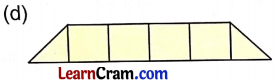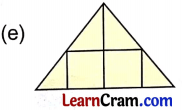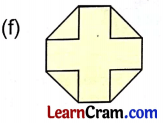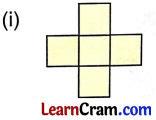# DAV Class 4 Maths Chapter 12 Worksheet 2 Solutions

The DAV Maths Book Class 4 Solutions and DAV Class 4 Maths Chapter 12 Worksheet 2 Solutions of Area offer comprehensive answers to textbook questions.

## DAV Class 4 Maths Ch 12 WS 2 Solutions

Question 1.
Find the area of these figures in terms of unit area (squares).4 squares5 squares13 squares5 squares4 squares7 squares4 squares